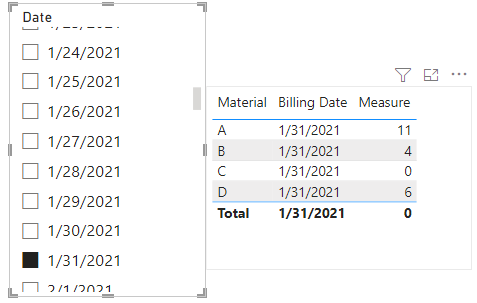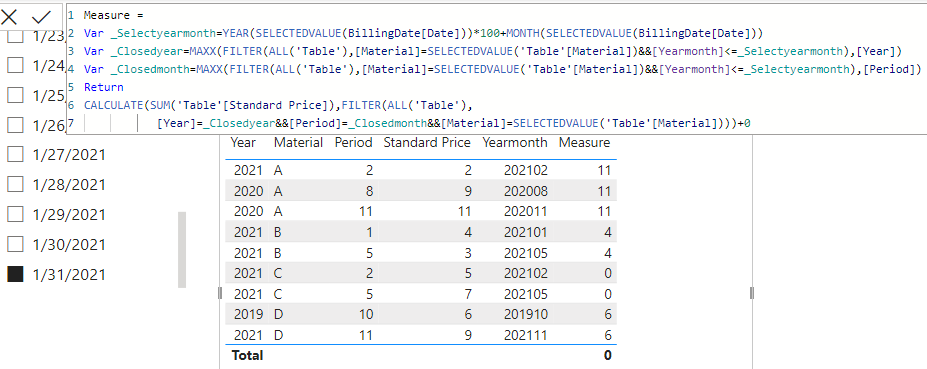cancel
Showing results for
Did you mean:Helper I

## Distinct search for a value based on validity date

Hi all,

I have a little challenge where I struggle with and hope to find help here in this great community. What is the challenge?  For a certain material I need to search for the standard price which is valid at a certain billing date.  My table contains  a Year column, a  Month column and a column with the standard price. E.g. if you have e.g. Year = 2020 and Month = 5, the record (standard price) is valid from 01.05.2020 and valid until there is a new record with a later date.. In my table there might be a valid entry for the needed month and year, but not necessarily. If not available I would like to have the standard price with the closest earlier record. E.g If I have billing date 31.01.2021 and  there are records for 02/2021, 11/2020 and 08/2020 available then I would like to have only the record from 11/2020 as it is the closest record  .  If there are only records available after 01/2021, then the expression should result in 0.

As you can see in the table below for Material A it should select the record where year is "2020" and month "11" for material B the record where year = "2021" and month = "1", for material C it should select 0 as the earliest record starts from Feb 2021 and hence after billing date "31.01.2021", for material D it should select the record where year = "2019" and month = "10"

The billing date is of cours dynamic.

Is there any smart way in doing this?

Br

Harry

 Material Year Period Standard Price A 2020 8 9 A 2020 11 11 A 2021 2 2 B 2021 1 4 B 2021 5 3 C 2021 2 5 C 2021 5 7 D 2019 10 6 D 2021 11 9
1 ACCEPTED SOLUTIONCommunity Support

Hi, @Harry1980

You can try the following methods.

``````Measure =
Var _Selectyearmonth=YEAR(SELECTEDVALUE(BillingDate[Date]))*100+MONTH(SELECTEDVALUE(BillingDate[Date]))
Var _Closedyearmonth=MAXX(FILTER(ALL('Table'),[Material]=SELECTEDVALUE('Table'[Material])&&[Yearmonth]<=_Selectyearmonth),[Yearmonth])
Var _Colsedvalue=CALCULATE(MAX('Table'[Standard Price]),FILTER(ALL('Table'),
[Yearmonth]=_Closedyearmonth&&[Material]=SELECTEDVALUE('Table'[Material])))+0
Var _Maxyearmonth=MAXX(FILTER(ALL('Table'),[Material]=SELECTEDVALUE('Table'[Material])),[Yearmonth])
Var _Maxvalue=CALCULATE(MAX('Table'[Standard Price]),FILTER(ALL('Table'),
[Yearmonth]=_Maxyearmonth&&[Material]=SELECTEDVALUE('Table'[Material])))+0
Return
IF(_Selectyearmonth>=_Maxyearmonth,_Maxvalue,_Colsedvalue)``````Is this the result you expect?

Best Regards,

Community Support Team _Charlotte

If this post helps, then please consider Accept it as the solution to help the other members find it more quickly.

4 REPLIES 4Helper I

Hi,

your amendment in the code seems to work😃. Thank you very much for your support in this. There is only one topic left which creates an issue. My data set shown here in the post was of course kept rather simple in order to simplify the challenge. However, you can imagine that our company data set contains a lot data points as we are selling thousands of different products. What I experience when using your code is that the computation power is not enough. Especially the code below seems to occupy a lot of processing power/memory.

```Var _Maxvalue=CALCULATE(MAX('Table'[Standard Price]),FILTER(ALL('Table'),
[Yearmonth]=_Maxyearmonth&&[Material]=SELECTEDVALUE('Table'[Material])))+0```

Hence, I am wondering whether there is a way to optimize/adjust the code in a way that it is requiring less ressources?

HarryCommunity Support

Hi, @Harry1980

You can try the following methods.
Measure:

``Yearmonth = SELECTEDVALUE('Table'[Year])*100+SELECTEDVALUE('Table'[Period])``
``````Measure =
Var _Selectyearmonth=YEAR(SELECTEDVALUE(BillingDate[Date]))*100+MONTH(SELECTEDVALUE(BillingDate[Date]))
Var _Closedyear=MAXX(FILTER(ALL('Table'),[Material]=SELECTEDVALUE('Table'[Material])&&[Yearmonth]<=_Selectyearmonth),[Year])
Var _Closedmonth=MAXX(FILTER(ALL('Table'),[Material]=SELECTEDVALUE('Table'[Material])&&[Yearmonth]<=_Selectyearmonth),[Period])
Return
CALCULATE(SUM('Table'[Standard Price]),FILTER(ALL('Table'),
[Year]=_Closedyear&&[Period]=_Closedmonth&&[Material]=SELECTEDVALUE('Table'[Material])))+0``````Is this the result you expect?

Best Regards,

Community Support Team _Charlotte

If this post helps, then please consider Accept it as the solution to help the other members find it more quickly.Helper I

Hi Charlotte,

thank you for your reply. Your suggestion does not exactly match what I would like to achieve. I see now that I should have provided more info.  I have created another way. It works fine as long as for the year a material was billed also a standard price  record exists/was created. For example for materials A-D the last record were created in 2021. Then my formula finds correct standard price until billing date 31.12.2021. But from 01.01.2022 the result is blank even though the last records from 2021 should still be valid for 2022 and 2023. For example for Material A the record valid from 02 2021 is the last available record and hence this would be still valid in case something was billed in 2022 or 2023. In this case it would be 2 for material

A in 2022 and 2023

Is there any smart way making it work also for 2022 or 2023?

Harry

used expression:

Before I have derived the code below I have added a month and a year column and have also increased the date range in table billing date (from 01.01.2019-31.12.2023) in order to be able to verify whether my code works

Valid Price =

VAR _targetmonth = max(BillingDate[Month])
VAR _targetyear = max(BillingDate[Year])
VAR _material = MAX('Table'[Material])
VAR _table =
FILTER(
'Table',
'Table'[Material]=_material
&&'Table'[Year] = _targetyear
&&'Table'[Period]<= _targetmonth
)

VAR _month =
MAXX(
_table,
'Table'[Period]
)
RETURN
MAXX(
FILTER(_table, 'Table'[Period]=_month),
'Table'[Standard Price]
)

Below is desired outcome for various billing dates
 Material Billing date Standard Price A 31.01.2023 2 B 31.01.2023 3 C 31.01.2023 7 D 31.01.2023 9 A 31.01.2021 11 B 31.01.2021 4 C 31.01.2021 0 D 31.01.2021 6 A 31.10.2019 0 B 31.10.2019 0 C 31.10.2019 0 D 31.10.2019 6Community Support

Hi, @Harry1980

You can try the following methods.

``````Measure =
Var _Selectyearmonth=YEAR(SELECTEDVALUE(BillingDate[Date]))*100+MONTH(SELECTEDVALUE(BillingDate[Date]))
Var _Closedyearmonth=MAXX(FILTER(ALL('Table'),[Material]=SELECTEDVALUE('Table'[Material])&&[Yearmonth]<=_Selectyearmonth),[Yearmonth])
Var _Colsedvalue=CALCULATE(MAX('Table'[Standard Price]),FILTER(ALL('Table'),
[Yearmonth]=_Closedyearmonth&&[Material]=SELECTEDVALUE('Table'[Material])))+0
Var _Maxyearmonth=MAXX(FILTER(ALL('Table'),[Material]=SELECTEDVALUE('Table'[Material])),[Yearmonth])
Var _Maxvalue=CALCULATE(MAX('Table'[Standard Price]),FILTER(ALL('Table'),
[Yearmonth]=_Maxyearmonth&&[Material]=SELECTEDVALUE('Table'[Material])))+0
Return
IF(_Selectyearmonth>=_Maxyearmonth,_Maxvalue,_Colsedvalue)``````Is this the result you expect?

Best Regards,

Community Support Team _Charlotte

If this post helps, then please consider Accept it as the solution to help the other members find it more quickly.